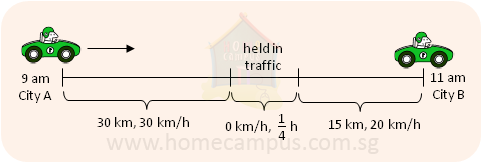## What is Average Speed?

Practice Unlimited Questions

#### Important Formulas:

Average speed  =
 Total distance travelled Total time taken

Total distance travelled  =  Average speed  ×  Total time taken

Total time taken  =
 Total distance travelled Average speed

#### 1. Understanding Average Speed

A car is going from City A to City B.
It starts from City A at 9:00 am and travels the first 30 km at 30 km/h.
Next, it is held in a traffic jam for
 1 4
h.
Finally, it travels the next 15 km at 20 km/h and arrives in City B at 11:00 am.a)   Does the car travel at the same speed throughout the journey?
No.

b)   What is the average speed of the car for the journey?
Total distance travelled by the car is 45 km.

Let's calculate the total time taken by the car to travel 45 km:
Time taken for the first 30 km  -->  1 h
Time lost in the traffic jam  -->
 1 4
h
Time taken for the next 15 km  -->
 3 4
h
Total time taken  =  1 h  +
 1 4
h  +
 3 4
h  =  2 h

The car travelled a total distance of 45 km in a total of 2 h.
It travelled an average of 22.5 km in 1 h.
Its average speed for the journey is 22.5 km/h.

#### 2. An aeroplane takes     5 1 2 hours to cover a distance of 4142 km. What is the average speed of the aeroplane?

Distance travelled  =  4142 km

Time taken  =
 5 1 2
h  =
 11 2
h

Average speed
=  4142 km  ÷
 11 2
h
=  4142 km  ×
 2 11
h
=  753.1 km/h

The average speed of the aeroplane is 753.1 km/h.

#### 3. Julie cycles at a speed of 215 m/min. How long does it take her to cycle a distance of 1500 m? Round off your answer to the nearest minute.

Speed  =  215 m/min

Distance travelled  =  1500 m

Time taken
=  1500 m  ÷ 215 m/min
=  6.97 min
≈  7 min

It takes her about 7 minutes.

#### 3. A roller skater skates at an average speed of 35 km/h. Find the distance he covers in 15 minutes.

Speed  =  35 km/h

Time taken  =  15 min  =
 1 4
h

Distance covered  =  35 m/h  ×
 1 4
h  =  8.75 km

He covers a distance of 8.75 km in 15 minutes.